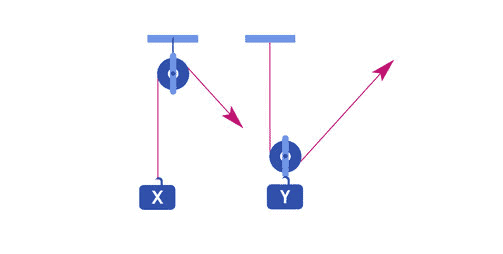# Pulleys

## Mechanical Aptitude Test – Pulleys

A pulley is defined as “A wheel with a grooved rim around which a cord passes. It acts to change the direction of a force applied to the cord and is chiefly used (typically in combination) to raise heavy weights”

When trying to figure out how to solve a pulley questions, it’s important to note that if the pulley is fixed (not moving), then the force required to pull the cord is equal to the weight. In the case that the pulley is not fixed, then the force is equal to half the weight. As an example, if you look at pulley X, the pulley is fixed. Therefore, if pulley X was given a weight of 20 pounds, it would require 20 pounds of force to lift the weight. If we have a look at pulley Y, the pulley is not fixed therefore, if the weight was 20 pounds, it would require 10 pounds of force to lift. (20 pounds / 2 = 10 pounds)Note: A good way to remember what to divide by is to count the number of ropes in the picture. In this example, if you look at the Y pulley, there are two ropes. If we where to add another pulley, there would be three ropes, therefore meaning you would have to divide by 3.

Find more pulley examples here.

[ CONTINUE TO ELECTRICAL CIRCUITS ]